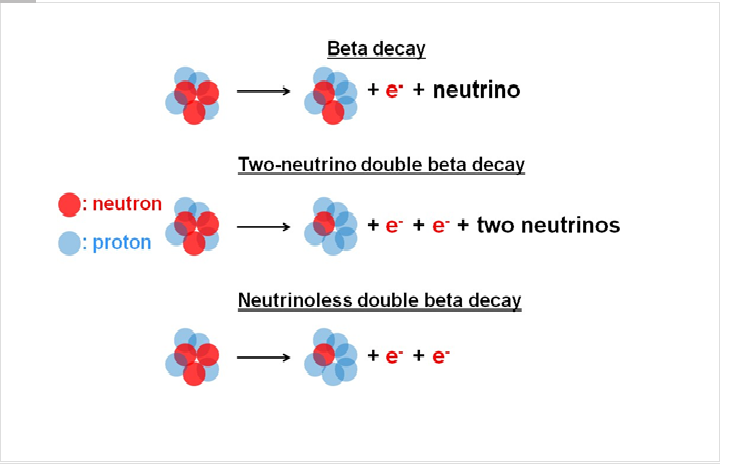# Double beta decays

The recent discovery of neutrino oscillations clearly indicates that neutrinos do have mass, which is compelling evidence of physics beyond the standard model of particle physics. However, while those observations provide information about the differences in the neutrino masses, they do not provide a direct measurement of the absolute mass scale and they leave un-answered questions about the properties of neutrinos.

To answer to those questions, the AMoRE experiment will explore one of the most sensitive searches for “neutrinoless double beta decay” in which only two electrons are emerged from the nuclear-beta decays. If this decay were observed, it would be a clear signal that neutrinos have a different mass structure than other elementary particles and would provide a measurement of the absolute neutrino mass scale.Figure 1: In the beta decay, one electron and one neutrino are emitted when a neutron is converted to a proton. In the two-neutrino double beta decay, two electrons are emitted with neutrinos when two neutrons are simultaneously converted to two protons. In neutrinoless double beta decay the two neutrinos are virtual and annihilate without being emitted.